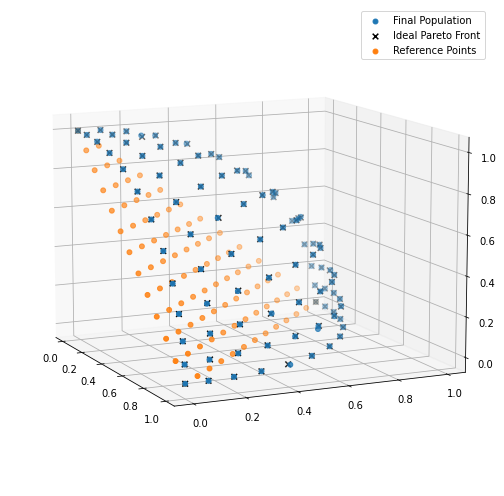[PYTHON] I implemented NSGA-Ⅲ, which is a multi-objective optimization problem.

• Articles sent by data scientists from the manufacturing industry
• This time, NSGA-Ⅲ was implemented (using sample code) in the multi-purpose optimization method.

Introduction

I will introduce the multi-purpose optimization (NSGA-Ⅲ) that I introduced the other day. What is NSGA-Ⅲ? Please refer to the above article for more information.

Library to use (deap)

I would like to implement this time using the genetic algorithm library deap.

Implementation of NSGA-Ⅲ

This time, there was a sample of NSGA-Ⅲ in the tutorial of deap, so I used that.

The python code is below.

#Import required libraries
from math import factorial
import random

import matplotlib.pyplot as plt
import numpy
import pymop.factory

from deap import algorithms
from deap import base
from deap.benchmarks.tools import igd
from deap import creator
from deap import tools

import matplotlib.pyplot as plt
import mpl_toolkits.mplot3d as Axes3d

First, we will design the genetic algorithm.

#Problem setting
PROBLEM = "dtlz2"
NOBJ = 3
K = 10
NDIM = NOBJ + K - 1
P = 12
H = factorial(NOBJ + P - 1) / (factorial(P) * factorial(NOBJ - 1))
BOUND_LOW, BOUND_UP = 0.0, 1.0
problem = pymop.factory.get_problem(PROBLEM, n_var=NDIM, n_obj=NOBJ)

#Algorithm parameters
MU = int(H + (4 - H % 4))
NGEN = 400
CXPB = 1.0
MUTPB = 1.0

# reference point
ref_points = tools.uniform_reference_points(NOBJ, P)

#Creating a goodness-of-fit class that is optimized by minimizing the goodness of fit
creator.create("FitnessMin", base.Fitness, weights=(-1.0,) * NOBJ)
#Create individual class Individual
creator.create("Individual", list, fitness=creator.FitnessMin)

#Gene generation function
def uniform(low, up, size=None):
try:
return [random.uniform(a, b) for a, b in zip(low, up)]
except TypeError:
return [random.uniform(a, b) for a, b in zip([low] * size, [up] * size)]

#Creating a Toolbox
toolbox = base.Toolbox()
#Functions that generate genes"attr_gene"Register
toolbox.register("attr_float", uniform, BOUND_LOW, BOUND_UP, NDIM)
#Function to generate an individual "individual""Register
toolbox.register("individual", tools.initIterate, creator.Individual, toolbox.attr_float)
#Functions that generate populations"population"Register
toolbox.register("population", tools.initRepeat, list, toolbox.individual)
#Evaluation function"evaluate"Register
toolbox.register("evaluate", problem.evaluate, return_values_of=["F"])
#Function to cross"mate"Register
toolbox.register("mate", tools.cxSimulatedBinaryBounded, low=BOUND_LOW, up=BOUND_UP, eta=30.0)
#Mutant function"mutate"Register
toolbox.register("mutate", tools.mutPolynomialBounded, low=BOUND_LOW, up=BOUND_UP, eta=20.0, indpb=1.0/NDIM)
#Individual selection method"select"Register
toolbox.register("select", tools.selNSGA3, ref_points=ref_points)

Since what is being done in each code is described so that it can be understood in the comment text, please forgive the part where the entire code is difficult to see.

This time, I am using the DTLZ2 problem (benchmark function).

Next is the actual evolutionary computation part.

def main(seed=None):
random.seed(1)

#Setting what to output to the log during the generation loop
stats = tools.Statistics(lambda ind: ind.fitness.values)
stats.register("avg", numpy.mean, axis=0)
stats.register("std", numpy.std, axis=0)
stats.register("min", numpy.min, axis=0)
stats.register("max", numpy.max, axis=0)

logbook = tools.Logbook()
logbook.header = "gen", "evals", "std", "min", "avg", "max"

#First generation generation
pop = toolbox.population(n=MU)
pop_init = pop[:]
invalid_ind = [ind for ind in pop if not ind.fitness.valid]
fitnesses = toolbox.map(toolbox.evaluate, invalid_ind)
for ind, fit in zip(invalid_ind, fitnesses):
ind.fitness.values = fit

record = stats.compile(pop)
logbook.record(gen=0, evals=len(invalid_ind), **record)
print(logbook.stream)

#Performing optimal calculations
for gen in range(1, NGEN):
#Population generation
offspring = algorithms.varAnd(pop, toolbox, CXPB, MUTPB)

invalid_ind = [ind for ind in offspring if not ind.fitness.valid]
#Goodness of fit calculation
fitnesses = toolbox.map(toolbox.evaluate, invalid_ind)
for ind, fit in zip(invalid_ind, fitnesses):
ind.fitness.values = fit

#Next generation selection
pop = toolbox.select(pop + offspring, MU)

record = stats.compile(pop)
logbook.record(gen=gen, evals=len(invalid_ind), **record)
print(logbook.stream)

return pop, pop_init, logbook

After that, call this main function.

if __name__ == "__main__":
pop, pop_init, stats = main()
pop_fit = numpy.array([ind.fitness.values for ind in pop])

pf = problem.pareto_front(ref_points)

This time, I would like to visualize the Reference Points and the results of the final generation.

fig = plt.figure(figsize=(7, 7))

p = numpy.array([ind.fitness.values for ind in pop])
ax.scatter(p[:, 0], p[:, 1], p[:, 2], marker="o", s=24, label="Final Population")

ax.scatter(pf[:, 0], pf[:, 1], pf[:, 2], marker="x", c="k", s=32, label="Ideal Pareto Front")

ref_points = tools.uniform_reference_points(NOBJ, P)

ax.scatter(ref_points[:, 0], ref_points[:, 1], ref_points[:, 2], marker="o", s=24, label="Reference Points")

ax.view_init(elev=11, azim=-25)
ax.autoscale(tight=True)
plt.legend()
plt.tight_layout()at the end

Thank you for reading to the end. This time, we confirmed the sample code for NSGA-Ⅲ, which is a multi-purpose optimization.

When using it in actual business, I think it will be difficult for both NSGA-II and NSGA-III to organize the constraints in detail according to the actual business situation. Also, if you try to find a Pareto solution with four or more objective variables, you cannot visualize it, so it seems necessary to devise that part as well.

If you have a request for correction, we would appreciate it if you could contact us.